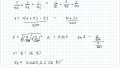# How do I find total impedance in a parallell circuit?

#### Aria Nemati

Joined Jul 19, 2019
27
What is the equation for equivalent resistance/impedance connected in parallel?
I have already tried this but didnt get the correct result. I gotZt should be 40 ohms not 0.025.

EDIT: I see I have done a mistake in calculating the fractions. I will recalculate and see if I get the right answer

Last edited:

#### Jony130

Joined Feb 17, 2009
5,435
Notice that the formula for Req clearly shows 1/Req = 1/Z1 + 1/Z2 ... therefore

Req = 1/Req = 1/0.025S = 40Ω

•Aria Nemati